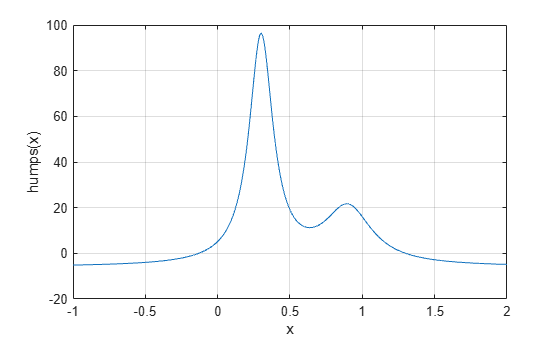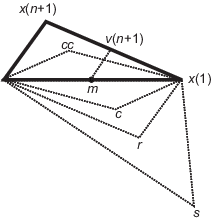## Optimizing Nonlinear Functions

### Minimizing Functions of One Variable

Given a mathematical function of a single variable, you can use the `fminbnd` function to find a local minimizer of the function in a given interval. For example, consider the `humps.m` function, which is provided with MATLAB®. The following figure shows the graph of `humps`.

```x = -1:.01:2; y = humps(x); plot(x,y) xlabel('x') ylabel('humps(x)') grid on```To find the minimum of the `humps` function in the range `(0.3,1)`, use

`x = fminbnd(@humps,0.3,1)`
```x = 0.6370 ```

You can see details of the solution process by using `optimset` to create options with the `Display` option set to `'iter'`. Pass the resulting options to `fminbnd`.

```options = optimset('Display','iter'); x = fminbnd(@humps,0.3,1,options)```
``` Func-count x f(x) Procedure 1 0.567376 12.9098 initial 2 0.732624 13.7746 golden 3 0.465248 25.1714 golden 4 0.644416 11.2693 parabolic 5 0.6413 11.2583 parabolic 6 0.637618 11.2529 parabolic 7 0.636985 11.2528 parabolic 8 0.637019 11.2528 parabolic 9 0.637052 11.2528 parabolic Optimization terminated: the current x satisfies the termination criteria using OPTIONS.TolX of 1.000000e-04 ```
```x = 0.6370 ```

The iterative display shows the current value of `x` and the function value at `f(x)` each time a function evaluation occurs. For `fminbnd`, one function evaluation corresponds to one iteration of the algorithm. The last column shows the procedure `fminbnd` uses at each iteration, a golden section search or a parabolic interpolation. For details, see Iterative Display.

### Minimizing Functions of Several Variables

The `fminsearch` function is similar to `fminbnd` except that it handles functions of many variables. Specify a starting vector x0 rather than a starting interval. `fminsearch` attempts to return a vector x that is a local minimizer of the mathematical function near this starting vector.

To try `fminsearch`, create a function `three_var` of three variables, `x`, `y`, and `z`.

```function b = three_var(v) x = v(1); y = v(2); z = v(3); b = x.^2 + 2.5*sin(y) - z^2*x^2*y^2;```

Now find a minimum for this function using `x = -0.6`, ```y = -1.2```, and `z = 0.135` as the starting values.

```v = [-0.6,-1.2,0.135]; a = fminsearch(@three_var,v) a = 0.0000 -1.5708 0.1803```

### Maximizing Functions

The `fminbnd` and `fminsearch` solvers attempt to minimize an objective function. If you have a maximization problem, that is, a problem of the form

`$\underset{x}{\mathrm{max}}f\left(x\right),$`

then define g(x) = –f(x), and minimize g.

For example, to find the maximum of tan(cos(x)) near x = 5, evaluate:

```[x fval] = fminbnd(@(x)-tan(cos(x)),3,8) x = 6.2832 fval = -1.5574```

The maximum is 1.5574 (the negative of the reported `fval`), and occurs at x = 6.2832. This answer is correct since, to five digits, the maximum is tan(1) = 1.5574, which occurs at x = 2π = 6.2832.

### `fminsearch` Algorithm

`fminsearch` uses the Nelder-Mead simplex algorithm as described in Lagarias et al. . This algorithm uses a simplex of n + 1 points for n-dimensional vectors x. The algorithm first makes a simplex around the initial guess x0 by adding 5% of each component x0(i) to x0. The algorithm uses these n vectors as elements of the simplex in addition to x0. (The algorithm uses 0.00025 as component i if x0(i) = 0.) Then, the algorithm modifies the simplex repeatedly according to the following procedure.

### Note

The keywords for the `fminsearch` iterative display appear in bold after the description of the step.

1. Let x(i) denote the list of points in the current simplex, i = 1,...,n+1.

2. Order the points in the simplex from lowest function value f(x(1)) to highest f(x(n+1)). At each step in the iteration, the algorithm discards the current worst point x(n+1), and accepts another point into the simplex. [Or, in the case of step 7 below, it changes all n points with values larger than f(x(1))].

3. Generate the reflected point

r = 2mx(n+1),

where

m = Σx(i)/n, i = 1...n,

and calculate f(r).

4. If f(x(1)) ≤ f(r) < f(x(n)), accept r and terminate this iteration. Reflect

5. If f(r) < f(x(1)), calculate the expansion point s

s = m + 2(mx(n+1)),

and calculate f(s).

1. If f(s) < f(r), accept s and terminate the iteration. Expand

2. Otherwise, accept r and terminate the iteration. Reflect

6. If f(r) ≥ f(x(n)), perform a contraction between m and the better of x(n+1) and r:

1. If f(r) < f(x(n+1)) (that is, r is better than x(n+1)), calculate

c = m + (rm)/2

and calculate f(c). If f(c) < f(r), accept c and terminate the iteration. Contract outside Otherwise, continue with Step 7 (Shrink).

2. If f(r) ≥ f(x(n+1)), calculate

cc = m + (x(n+1) – m)/2

and calculate f(cc). If f(cc) < f(x(n+1)), accept cc and terminate the iteration. Contract inside Otherwise, continue with Step 7 (Shrink).

7. Calculate the n points

v(i) = x(1) + (x(i) – x(1))/2

and calculate f(v(i)), i = 2,...,n+1. The simplex at the next iteration is x(1), v(2),...,v(n+1). Shrink

The following figure shows the points that `fminsearch` can calculate in the procedure, along with each possible new simplex. The original simplex has a bold outline. The iterations proceed until they meet a stopping criterion.### Reference

 Lagarias, J. C., J. A. Reeds, M. H. Wright, and P. E. Wright. “Convergence Properties of the Nelder-Mead Simplex Method in Low Dimensions.” SIAM Journal of Optimization, Vol. 9, Number 1, 1998, pp. 112–147.